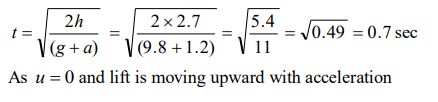## Motion in a Straight Line Questions and Answers Part-6

1. The position of a particle moving in the xy-plane at any time t is given by $X=\left(3t^{2}-6t\right)$  metres, $Y=\left(t^{2}-2t\right)$  metres. Select the correct statement about the moving particle from the following
a) The acceleration of the particle is zero at $t=0$  second
b) The velocity of the particle is zero at $t=0$  second
c) The velocity of the particle is zero at $t=1$  second
d) The velocity and acceleration of the particle are never zero

Explanation:2. If body having initial velocity zero is moving with uniform acceleration $8 m\diagup sec^{2}$  the distance travelled by it in fifth second will be
a) 36 metres
b) 40 metres
c) 100 metres
d) Zero

Explanation: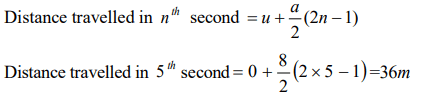3. An alpha particle enters a hollow tube of 4 m length with an initial speed of 1 km/s. It is accelerated in the tube and comes out of it with a speed of 9 km/s. The time for which it remains inside the tube is
a) $8\times 10^{-3}s$
b) $80\times 10^{-3}s$
c) $800\times 10^{-3}s$
d) $8\times 10^{-4}s$

Explanation: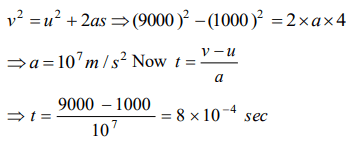4. Two cars A and B are travelling in the same direction with velocities $V_{1}$ and $V_{2}\left(V_{1}>V_{2}\right)$   . When the car A is at a distance d ahead of the car B , the driver of the car A applied the brake producing a uniform retardation a . There will be no collision when
a) $d<\frac{\left(V_{1}-V_{2}\right)^{2}}{2a}$
b) $d<\frac{V_{1}^{2}-V_{2}^{2}}{2a}$
c) $d>\frac{\left(V_{1}-V_{2}\right)^{2}}{2a}$
d) $d>\frac{V_{1}^{2}-V_{2}^{2}}{2a}$

Explanation: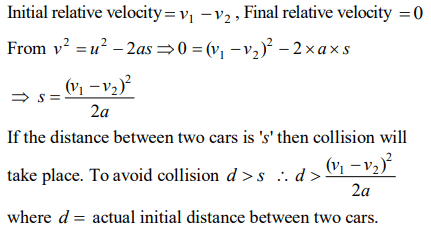5. A body of mass 10 kg is moving with a constant velocity of 10 m/s. When a constant force acts for 4 seconds on it, it moves with a velocity 2 m/sec in the opposite direction. The acceleration produced in it is
a) $3m\diagup sec^{2}$
b) $-3m\diagup sec^{2}$
c) $0.3m\diagup sec^{2}$
d) $-0.3m\diagup sec^{2}$

Explanation:6. A body starts from rest from the origin with an acceleration of $6m\diagup sec^{2}$  along the x-axis and $8m\diagup sec^{2}$  along the y-axis. Its distance from the origin after 4 seconds will be
a) 56 m
b) 64 m
c) 80 m
d) 128 m

Explanation: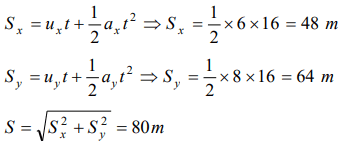7. A car moving with a velocity of 10 m/s can be stopped by the application of a constant force F in a distance of 20 m. If the velocity of the car is 30 m/s, it can be stopped by this force in
a) $\frac{20}{3}m$
b) 20 m
c) 60 m
d) 180 m

Explanation: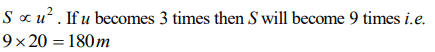8. The displacement of a particle is given by $y=a+bt+ct^{2}-dt^{4}$    . The initial velocity and acceleration are respectively
a) $b,-4d$
b) $-b,2c$
c) $b,2c$
d) $2c,-4d$

Explanation:9. A car moving with a speed of 40 km/h can be stopped by applying brakes after atleast 2 m. If the same car is moving with a speed of 80 km/h, what is the minimum stopping distance
a) 8 m
b) 2 m
c) 4 m
d) 6 m10. An elevator car, whose floor to ceiling distance is equal to 2.7 m, starts ascending with constant acceleration of $1.2 m s^{-2}$ . 2 sec after the start, a bolt begins fallings from the ceiling of the car. The free fall time of the bolt is
a) $\sqrt{0.54 s}$
b) $\sqrt{6 s}$